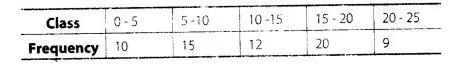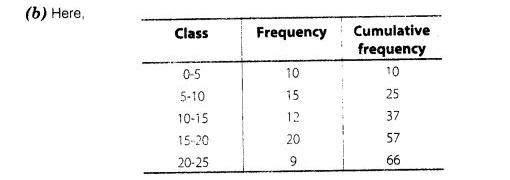# For the following distribution,

Question:

For the following distribution,the sum of lower limits of the median class and modal class is

(a) 15

(b) 25

(c) 30

(d) 35

Solution:Now, $\frac{N}{2}=\frac{66}{2}=33$, which lies in the interval $10-15$. Therefore, lower limit of the median class is

10.                                              .         ,
The highest frequency is 20, which lies in the interval 15-20. Therefore, lower limit of modal class is 15. Hence, required sum is 10 + 15 = 25.